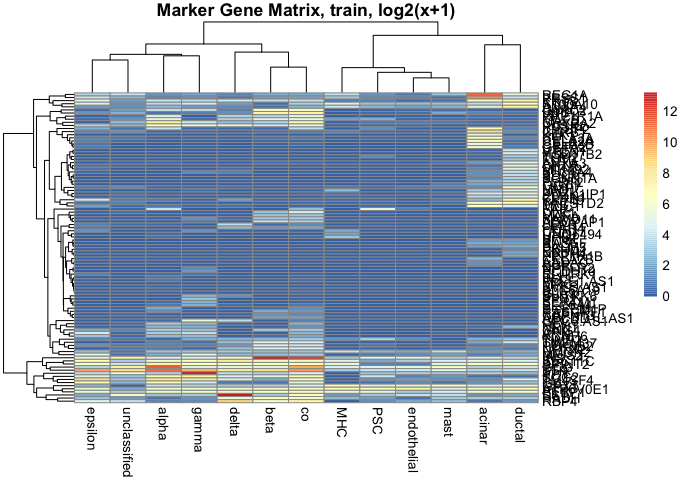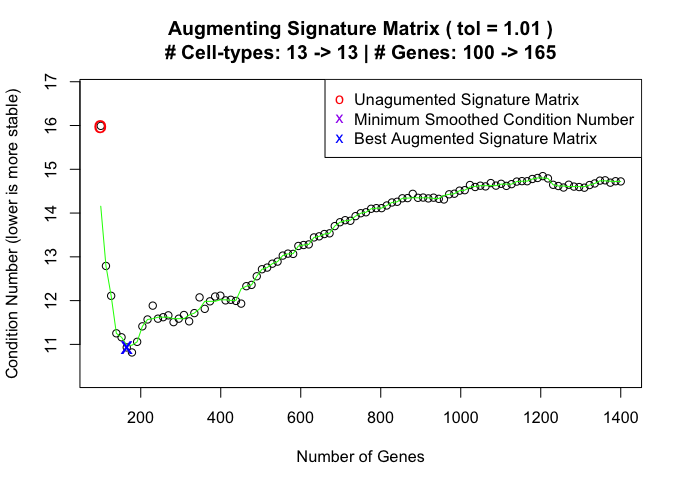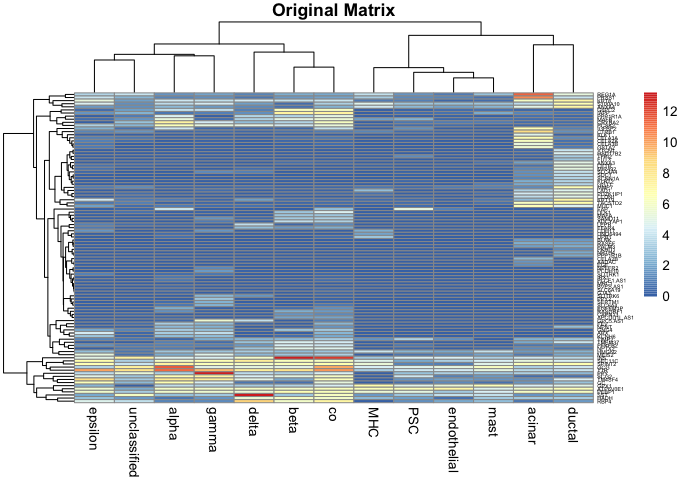install.packages(‘devtools’)

### Step 1: Build signature matrices from the normal data and estimate the accuracy on the diabetes data

All the data comes from E-MTAB-5061 - Single-cell RNA-seq analysis of human pancreas. In this vignette,instead of splitting the normal data into training and test set, all the signature matrices are built using the entire normal data, and then tested on the new diabetes data.

``````normalData <- log(ADAPTSdata3::normalData.5061+1)

#### Step 1a: Build a deconvolution seed matrix using ranger forest and estimate the accuracy on pseudo bulk diabetes

ADAPTS provides the option of building a new seed matrix de novo based on the sample given, in addition to augmenting existing signature matrices, such as LM22. This is particularly helpful for single cell data sets, where the cell types present have come from their native tissue.

``````trainSet.30sam <- ADAPTS::scSample(RNAcounts = normalData, groupSize = 30, randomize = TRUE)
trainSet.3sam <- ADAPTS::scSample(RNAcounts = normalData, groupSize = 3, randomize = TRUE)

seedMat<-ADAPTS::buildSeed(trainSet=normalData, trainSet.3sam =trainSet.3sam, trainSet.30sam = trainSet.30sam, genesInSeed = 100, groupSize = 30, randomize = TRUE, num.trees = 1000, plotIt = TRUE)  ````````````pseudobulk.test <- data.frame(test=rowSums(diabetesData))
pseudobulk.test.counts<-table(sub('\\..*','',colnames(diabetesData)))
actFrac.test <- 100 * pseudobulk.test.counts / sum(pseudobulk.test.counts)

colnames(estimates.test)<-'seed'

estimates.test\$actFrac<-round(actFrac.test[rownames(estimates.test)],2)

#### Step 1b: Build a deconvolution matrix using all the genes and estimate the accuracy on pseudo bulk diabetes data

This step tests if building signature matrix is really necessary by comparing the performance of signature matrices and all-gene matrix.

``````allGeneSig <- apply(trainSet.3sam, 1, function(x){tapply(x, colnames(trainSet.3sam), mean, na.rm=TRUE)})

colnames(estimates.allGene)<-'all'

estimates.test<-cbind(estimates.allGene,estimates.test)

``````gList <- ADAPTS::gListFromRF(trainSet=trainSet.30sam)